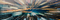Source: Unsplash

# Obtaining Top Neural Network Performance Without Any Training

## We know less about NNs than we thought

How do neural networks generalize when they are so overparametrized?

As we try to answer this question with recent research, we will find that we know much less about neural networks than we thought, and understand why a random initialized network can perform just as well as a trained one.

In more standard machine learning practice, it’s conventional to minimize the number of parameters in a model to prevent overfitting and ensure true learning instead of memorization. On the other hand, machine learning engineers simply keep on stuffing neural networks to become larger and larger, which somehow works. This violates what should be common sense.

One should not increase, beyond what is necessary, the number of entities required to explain anything.
- Occam’s Razor

It’s not uncommon for modern neural networks to achieve 99.9 percent or even 100 percent accuracy on the training set — which usually would be a warning of overfitting. Surprisingly, however, neural networks can achieve similarly high test set scores.

One common answer proposed as to why neural networks don’t overfit is the role of regularization. Unfortunately, this isn’t the case — in a study conducted by Zhang et al., an Inception architecture without various regularization methods didn’t perform much worse than one with regularization. Thus, one cannot argue that regularization is the basis for generalization.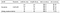Source: Zhang et al. Image free to share.

Neural network pruning offers a glimpse into one convincing answer, however, which certainly shifts perspectives on how neural networks work.

With neural network pruning, over 90 percent — in some cases 95 or even 99 percent — of neural network weights and neurons can be eliminated with little to no loss on performance. This begs the question: if a network can perform equivalently with only 5 percent of the weights, what purpose does the remainder 95 percent actually serve?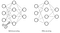Source: TensorFlow. Image free to share.

This gives one partial answer to the generalization question — neural networks are overparametrized, but somehow don’t use the majority of the weights. Instead, a few weights carry most of the information and the rest serve as some combination of noise and passive components. Perhaps they store memorized information only pertaining to the training set (neural networks can obtain perfect accuracy with completely random labels).

Luckily, this idea has been formalized as the Lottery Ticket Hypothesis. Simply put, a neural network is a massive random lottery — weights are randomly initialized. As the optimizer determines how to update certain weights considering their current values, certain subnetworks are delegated to carry most of the information flow simply because their initialized weights were the right values to spark growth.

These subnetworks are said to be ‘winning tickets’. Analyzing neural networks through lens of the Lottery Ticket Hypothesis answers many questions about neural networks and deep learning:

• Q: Why do larger neural networks generally perform better?
A: They operate larger lotteries with more connections and hence have a higher chance of finding successful winning tickets.
• Q: Why does training directly from sparse networks perform worse than first training from a large dense network and pruning?
A: There are more possible subnetworks for the neural network to explore. The eventual goal of a neural network is to find an optimal sparse subnetwork, but it cannot begin with one.
• Q: Why does initializing all weights to 0 not perform well?
A: Each subnetwork is the same, and hence each is individually weak. There is no initial diversity for the optimizer to identify and grow out strong subnetworks (winning tickets).
• Q: Why can overparametrized, massive neural networks still learn?
A: Only a segment of the neural network — the winning tickets — provide the generalization ‘learning’ component. These are identified and developed by the optimizer because they begin at convenient values.

The authors provide a formal definition:

A randomly-initialized, dense neural network contains a subnetwork that is initialized such that — when trained in isolation — it can match the test accuracy of the original network after training for at most the same number of iterations.

Rephrased, if one were to take only the subnetwork and train it, the performance would match or beat the original network under the same training conditions.

In order to find these tickets, the authors propose Iterative Magnitude Pruning (IMP), which operates by the following steps:

1. Begin with a dense initialization and train the network.
2. Determine the s% smallest magnitude weights (these are the weights that don’t have important values and hence contain little information).
3. Create a binary mask (multiply by 1 to keep, multiply by 0 to discard) to prune these weights.
4. Retrain the sparse network with its previous initial weights (retrain the network with the same weights, but with the mask such that some of the weights no longer have an input in the process).
5. Repeat the pruning process (steps 2–4) until the desired sparsity is reached or the test error has gotten too large.

This idea, however, can be taken even further. Ramanujan et al. found that within a randomly weighted Wide ResNet 50 lay a randomly weighted subnetwork smaller than but matching in performance with a ResNet 34 trained on ResNet. Initially, networks contain subnetworks that can achieve impressive performance without its weights ever touching data.

The researchers propose a complimentary conjecture to the Lottery Ticket Hypothesis: “within a sufficiently overparameterized neural network with random weights (e.g. at initialization), there exists a subnetwork that achieves competitive accuracy”.

An ‘edge-popup’ algorithm is used to find these randomly weighted winning tickets. While each edge (connection)’s value remains unchanged, it is associated with a score (that may change).

Each iteration, the edges corresponding to the top k% of scores are selected and the rest pruned. Based on the performance of this sparse subnetwork, stochastic gradient descent is used to update all of the scores optimally; this allows for edges that have been pruned mistakenly to come back in the future.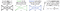Source: Ramanujan et al. Image free to share.

Essentially, this pruning technique is the same as how one would train a regular neural network; the difference is that scores, which determine the architecture of a network, instead of weights, are tuned. Using this algorithm, random subnetworks can be chosen in a manner so effective that the performance is comparable with top-performing architectures.

As intuition, the authors offer an example — consider an untrained neural network `N` with an arbitrary width (the number of channels in the case of CNNs) with weights initialized to a normal distribution (standard procedures like Xavier or Kaiming). Let `τ` be a network of the same architecture trained such that it attains good performance.

Let `q` be the probability that a subnetwork of `N` has weights that are close enough to `τ` to obtain the same (or better) performance. Instinctively, `q` is very small, but it is regardless not zero (it is possible). Hence, the probability that a subnetwork of `N` does not obtain similar performance to `τ` is (1−`q`), which is fairly large.

The probability, on the other hand, that no subnetwork of `N` obtains a similar performance to `τ` is (1−`q`)ˢ, where s is the number of subnetworks. This is because each subnetwork must fail to obtain similar performance to `τ`. As any simple calculator experiment will yield, raising fractions — even high ones like 0.999999 — to high exponents will result in arbitrarily small values.

Thus, as s increases with the width and depth of the network, the chance that a subnetwork will perform just as well as a well-trained network increases drastically, given that each subnetwork is unique. This is an extension upon reasoning based in the Lottery Ticket Hypothesis.

The authors experimented with convolutional neural networks of different depths on the CIFAR-10 dataset. Blue and orange lines represent the learned weights of Adam and SGD optimizers, whereas blue and red lines represent the accuracy of random subnetworks of different initialization methods, across the percent of top-scoring weights kept (x-axis, this is k in the edge popup algorithm).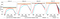Source: Ramanujan et al.

Note that as the depth of the network increases, a subnetwork with random weights actually performs better than a trained network. From earlier discussed intuition, this at least makes a bit of sense, and undoubtedly shakes up how we view the value and process of neural network training.

Practically, this fascinating finding has little value, but it reveals the need to explore more into the key factors driving learning in neural networks.

These recent advances in pruning, the Lottery Ticket Hypothesis, the finding of top-performing weights, and model compression are unravelling large parts of the blackbox nature of neural networks that have too long been ignored. With these findings, researchers may be able to find and develop even more efficient optimization and learning methods.

## Summary

• The success of neural network pruning, among other studies that debunk the idea that regularization is at the root of generalization, point to an important question as to exactly how and why neural networks appear to generalize while simultaneously appearing to overfit.
• The Lottery Ticket Hypothesis states that neural networks are really running large lotteries where subnetworks initialized to convenient values are indirectly chosen and developed by the optimizer to process most of the information flow.
• In a sufficiently large untrained and randomly initialized network, one can find a subnetwork with random weights that performs as well as an unpruned, trained network.

## Recommended Reading

Thanks for reading!